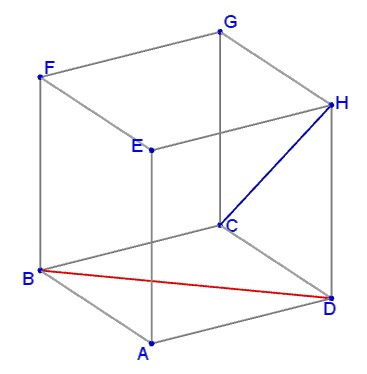# Angles in a Cube I

### What Is That About?### Problem

Let denote the vertices of a cube $ABCD$ (for the bottom face) and $EFGH$ (for the top face), with $E$ above $A,$ etc.What is the angle between two lines, $CH$ and $BD?$

### Solution

Start with drawing $HF\parallel BD.$ $\widehat{(BD,CH)}=\widehat{(HF,CH)}.$ Add $CH.$Now observe that $HF=CF=CH$ as all three are the diagonals of the faces of a cube. Thus $\Delta BCH$ is equilateral and $\angle CFH=\widehat{(HF,CH)}=60^{\circ}.$ It follows that $\widehat{(BD,CH)}=60^{\circ}.$### Related materialRead more...

• Angles in a Cube II
• Angles in a Cube III
• Bimedians in a Regular Tetrahedron
• Cut the Cone
• Cut the Cube
• Cut the Cylinder
•Copyright © 1996-2018 Alexander Bogomolny

 65256271